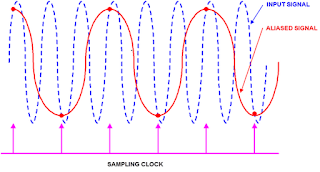## You need to test, we're here to help.

You need to test, we're here to help.

## 10 June 2013

### Oscilloscope Basics: Sampling Rate

In a recent overview post on oscilloscope banner specifications,
one of the topics covered is sampling rate. Let's do a somewhat deeper dive on that topic and look at what sampling rate means to oscilloscope users.

Sampling rate is a complex subject as it is tied closely to two other instrument parameters: bandwidth and acquisition memory depth. Sampling rate in and of itself is, of course, explained fundamentally by Nyquist's sampling theorem, which tells us:
For a limited bandwidth signal with a maximum frequency fmax, the equally-spaced sampling frequency fs must be greater than twice the maximum frequency fmax to have the signal be uniquely reconstructed without aliasing.
So from Nyquist's theorem, we see that the sampling frequency must be at least twice the highest frequency component in the input signal to be able to reproduce the signal on the oscilloscope screen without distortion.

An examination of how sampling rate affects the uncertainty in time-domain measurements would tell us that there is a point of diminishing return. Consider the case of a typical timing measurement, characterizing the period @ level of a square wave (Figure 1). The standard deviation of that period @ level measurement would give us the RMS jitter value of the period of our square wave. We would see continuous improvement of that RMS jitter value out to an oversampling rate (sample rate\bandwidth) of perhaps 2.5 times the Nyquist rate of 2fmax, indicating improvement in the measurement certainty. But by the time we're oversampling at perhaps four to five times the Nyquist rate, we see negligible improvement at best.Figure 1: Shown is an example of aliasing that results from a sampling rate of less than the Nyquist frequency of 2fmax.
Should one attempt to sample a signal at less than twice per cycle, the oscilloscope is apt to interpret the signal as having a lower frequency than it really does. This is what leads to aliasing (Figure 1). The frequency of the alias is the difference frequency between the input signal and the sampling frequency (or one of its harmonics). The aliased signal will also appear to be poorly triggered and to move horizontally.

The depth of a given oscilloscope's acquisition memory is another factor to consider in determining what sampling rate gives you the biggest bang for your buck. The relationship can be described in high-level terms by saying that for a given sampling rate, the size of the buffer memory determines how long it can capture a signal before the memory is full. An oscilloscope with a high sampling rate and small acquisition memory will only be capable of using its full sampling rate on the top few timebase settings. Compensating with a lower sampling rate to capture a longer waveform risks compromising the fidelity of the signal acquisition.